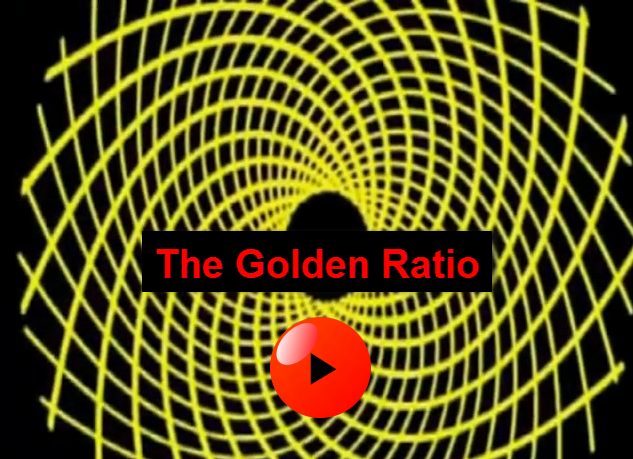# Pythagoras, Sacred GeometryPythagoras, Sacred Geometry, "The Fundamental Programming Language of the Holographic Universe"

This is the biggest mystery. For over 25,000 years the Universal Truth was not available to the people of this planetary system. It is now finally being received on this planet. Deep seekers of the truth that are open minded and ready will receive it.

Throughout time there has been intersecting moments when the cycles of the great cosmic clocks windup in such a way that we are not only given a gimps of all that is possible, but we actually have the opportunity to step beyond all what we've known as truth and participate in a grander truth within a higher level of consciousness.

# The Fibonacci Sequence Explained

The Fibonacci sequence is a set of numbers that starts with a one or a zero, followed by a one, and proceeds based on the rule that each number (called a Fibonacci number) is equal to the sum of the preceding two numbers. If the Fibonacci sequence is denoted F (n), where n is the first term in the sequence, the following equation obtains for n = 0, where the first two terms are defined as 0 and 1 by convention:

F (0) = 0, 1, 1, 2, 3, 5, 8, 13, 21, 34 ...

In some texts, it is customary to use n = 1. In that case, the first two terms are defined as 1 and 1 by default, and therefore:

F (1) = 1, 1, 2, 3, 5, 8, 13, 21, 34 ...

The Fibonacci sequence is named for Leonardo Pisano (also known as Leonardo Pisano or Fibonacci), an Italian mathematician who lived from 1170 - 1250. Fibonacci used the arithmetic series to illustrate a problem based on a pair of breeding rabbits:

"How many pairs of rabbits will be produced in a year, beginning with a single pair, if in every month each pair bears a new pair which becomes productive from the second month on?" The result can be expressed numerically as: 1, 1, 2, 3, 5, 8, 13, 21, 34 ...

Fibonacci numbers are of interest to biologists and physicists because they are frequently observed in various natural objects and phenomena. The branching patterns in trees and leaves, for example, and the distribution of seeds in a raspberry are based on Fibonacci numbers.

# Gods Fingerprint→ The Fibonacci Sequence – Golden Ratio and The Fractal Nature of Reality"Fibonacci numbers are of interest to biologists and physicists because they are frequently observed in various natural objects and phenomena. The branching patterns in trees and leaves, for example, and the distribution of seeds in a raspberry are based on Fibonacci numbers. A Sanskrit grammarian, Pingala, is credited with the first mention of the sequence of numbers, sometime between the fifth century B.C. and the second or third century A.D. Since Fibonacci introduced the series to Western civilization, it has had a high profile from time to time. Recently, in The Da Vinci Code , for example, the Fibonacci sequence is part of an important clue." whatis.techtarget.com/definiti...

# Why is 1.618034 So Important?Imagine if there was a perfect number, a single number so flawless it formed the basis for all art and music; a number so important that it could be used across the disciplines of mathematics and physics, and a number so profoundly purposeful that the natural world and the universe would bend to its whims. The Fibonacci Sequence is a string of numbers that begins with zero and one which can be extended infinitely by adding up any two consecutive numbers to find the next in the sequence. The Golden Ratio is a mathematical term used to describe the relationship of two figures, whereby the numbers seem to be in some form of complimentary ratio.To link the Fibonacci Sequence and the Golden Ratio we must look at the ratio between two consecutive numbers in Fibonacci, and observe the pattern this ratio takes as you use ever higher numbers. The Golden Ratio has fascinated the world's most knowledgeable people for millennia since its discovery, and we have seen its influence in some of the finest works mankind has ever produced. Is the Golden Ratio a universal law applied by nature? The evidence seems to suggest so, and the aforementioned Leonardo Da Vinci may have alluded to this in some of his most famous works.